• Submissions# CFOA based Integrator Suitable for Analog Signal Processing

Praween K Sinha*, Mohit Kumar, Gautam Kunal Haruray and Neelam Sharma

Department of Electronics and Communication Engineering, Maharaja Agrasen Institute of Technology, India

*Corresponding author: Praween K Sinha, Department of Electronics and Communication Engineering, Maharaja Agrasen Institute of Technology, Sec-22, Rohini, New Delhi-110086, India

Submission: August 25, 2018;Published: October 30, 2018

DOI: 10.31031/COJEC.2018.01.000513ISSN 2640-9739
Volume1 Issue3

#### Abstract

Current Feedback Operational Amplifier (AD844) uses a circuit design that emphasizes current-mode operation, which is inherently much faster than voltage-mode operation because it is less effected by stray node-capacitances. When fabricated using high-speed complementary bipolar processes, CFOA’s can be orders of magnitude faster than other available feedback amplifiers ex. VFA’s. With CFOAs, the amplifier gain may be controlled independently of bandwidth. All these constitutes the major advantages of CFOAs. Some new and more efficient active RC integrator circuit realizations, using minimum passive components grounded and a current feedback operational amplifier (CFOA) device are proposed. Integrator with Grounded passive components allow better usability in VLSI. Finally, experimental result by wave processing has been verified using Proteus software.

Keywords: Integrators; Current-feedback-operational-amplifiers; Current mode circuits

#### Introduction

Active - RC integrator circuit is widely used in analog computers, analog-to-digital converters and wave-shaping circuits. These circuits essentially fuse a ratio type (y1/y2) function involving an active device like the voltage operational amplifier, operational trans-conductance amplifier, current conveyor, and current feedback operational amplifiers [1-6]. But use of Current Feedback Operational Amplifier’s has increased exponentially in past few years [3-5] because of the its distinctive characteristics, in comparison with traditional operational amplifiers, like inverting input with low input resistance, additional output with high output resistance, frequency range extension, and very fast large signal response (major advantages over the op-amp are the increased device bandwidth at higher slew-rate, and, accurate port tracking properties leading to a less sensitive design) [7-13]. Typically, these amplifiers perform on the complementary bipolar technology on heterogeneous symmetric p-n-p and n-p-n transistors. Experimental results on wave processing have been verified with Proteus simulation.

#### CFOA

Figure 1:Current feedback operational amplifier (AD844).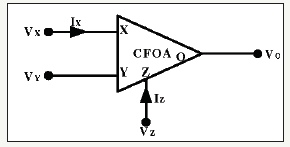The current feedback operational amplifier (CFOA) is a type of electronic amplifier which have current as inverting input, rather than voltage as in a conventional voltage-feedback operational amplifier (VFA). Figure 1 gives a representation of the amplifier with potential (Y) and current (X) inputs and potential (O) and current (Z) outputs. A CFOA is a four terminal building block characterized by the following terminal equations.These circuits showcase better performance, particularly higher speed and better bandwidth, than voltage mode operational amplifiers (VOA). The current feedback operational amplifier (CFOA) close-loop bandwidth is independent of its close-loop gain (provided that the feedback resistance is kept constant and much higher than the CFOA inverting input resistance) unlike VOAbased circuits, which are limited by a constant gain-bandwidth product. The CFOA block is preferred for low-voltage, low-power applications and is characterized by low voltage-transfer errors and high output driving current capability .

In this paper, new circuit models are developed by giving appropriate basic thought to the subject all in current mode. As a result, new integrator circuits have been developed having efficient performance and characteristics. New sets of circuit models based on current mode approach, which have been developed are likely to find productive use in the field of modern electronics. This work offers some circuits having improved characteristics with respect to tenability, component count, integrability i.e. chip area economization. These circuits can be of great value in signal processing, communication and instrumentation area.

### Mathematical Analysis

Integrator: Figure 2

Figure 2:integrator.Integrator 1: Figure 3

Figure 3:Integrator 1.Integrator 2: Figure 4

Figure 4:Integrator 2.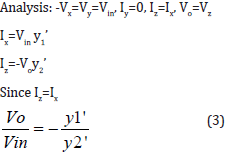### Case I (Integrator 1) Figure 5

Figure 5:Schematic diagram case I.Figure 6:Simulation of case I.Wave conversion at 10Hz by CFOA of Figure 5 using R=10KΩ, C=9uF

### Case II (Integrator 2) Figure 7Figure 7:Schematic of case II.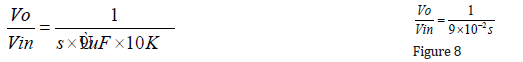Figure 8:Simulation of case II.Case III (Integrator) Figure 9Figure 9:Schematic of case III.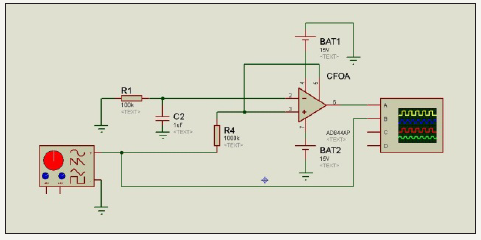Figure 10:Simulation of case III.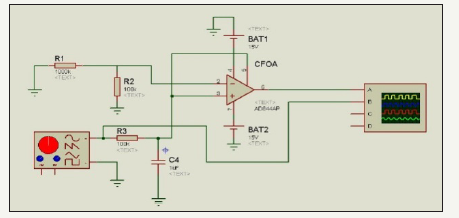Wave conversion at 10Hz by CFOA of Figure 7 using R=10KΩ, C=9uF.

Wave conversion at 10Hz by CFOA of Figure 9 using R1=100kΩ, R4=100kΩ

Case IV (Integrator 2) Figure 11Figure 11:Schematic of case IV.Figure 12:Simulation of case IV.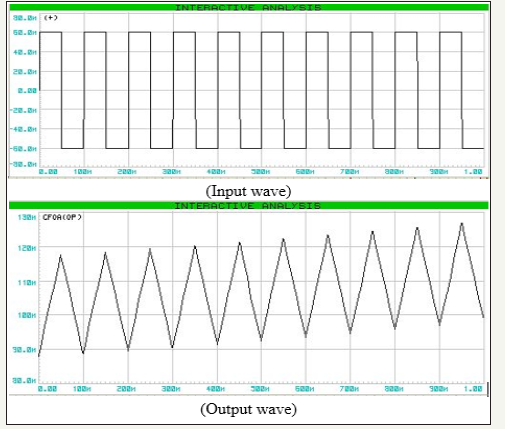Wave conversion at 10 Hz by CFOA of Figure 11 using R1=1000kΩ, R2=100kΩ, R3=100kΩ , C4=1uf

Case V (Integrator 3) Figure 13Figure 13:Simulation of case V.Figure 14:Simulation of case V.Figure 14

Wave conversion at 10Hz by CFOA of Figure13 using C1=1pF, R3=1200kΩ, C4=1uf.

Figure 15:Simulation of case VI.### Case VI (Integrator 4) Figure 15Figure 16:Simulation of case VI.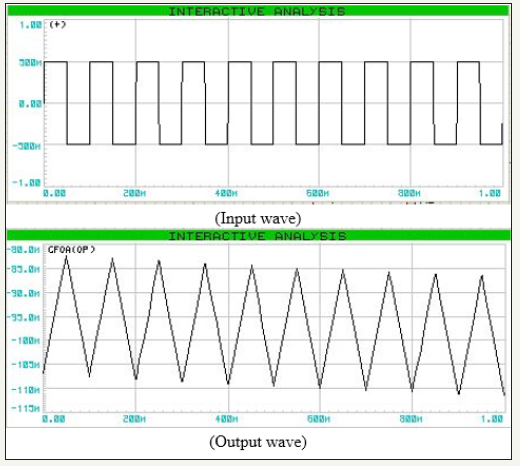Wave conversion at 10Hz by CFOA of Figure15 using When C1=1pF, R3=1200kΩ, C4=1uf, R2=1000kΩ

#### Experimental Result

All the proposed configurations had been tested with Proteus simulation. The AD-844 CFOA was used as the active device. In our experiments, regulated bias voltages were set at 0±12Vdc. for the CFOA. Time domain tests for wave conversion measurement were carried out. Some typical results on wave conversion by the integration functions are shown in Table 1. For these tests, the input signals were square wave for the integrators. The gain and frequency had been measured from the oscilloscope display on Proteus simulation. The proposed circuits exhibited satisfactory response practically expected from an integrator.

Table 1:Results (Practical and theoretical values).#### Conclusion

In this paper, an analysis and simulation is presented about six new cases of active RC integrator circuit realizations, using minimum passive components grounded and a current feedback operational amplifier (CFOA) device are proposed. Integrator with grounded passive components allows better usability in VLSI. The realizability equations are derived and experimental result by wave processing has been verified using Proteus software. The proposed circuits exhibited satisfactory response as practically expected from an integrator.

We at Crimson Publishing are a group of people with a combined passion for science and research, who wants to bring to the world a unified platform where all scientific know-how is available read more...